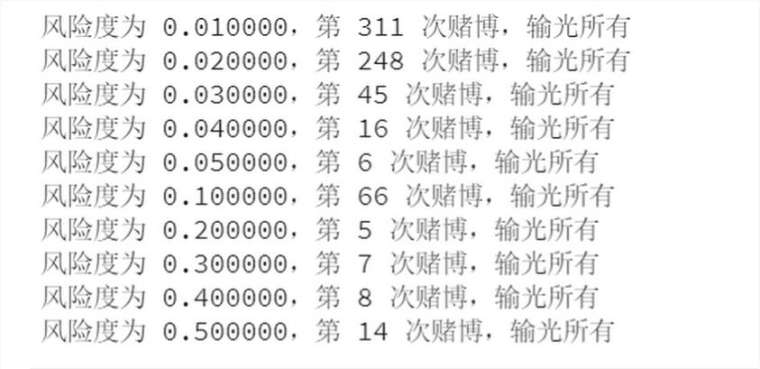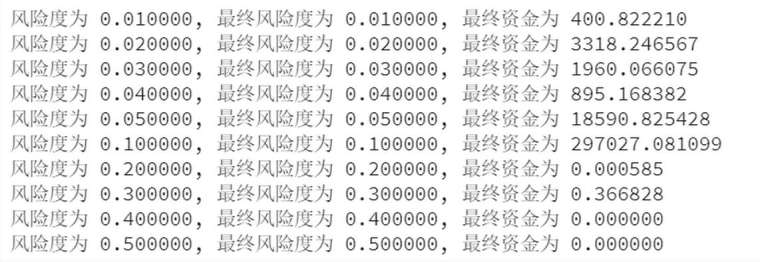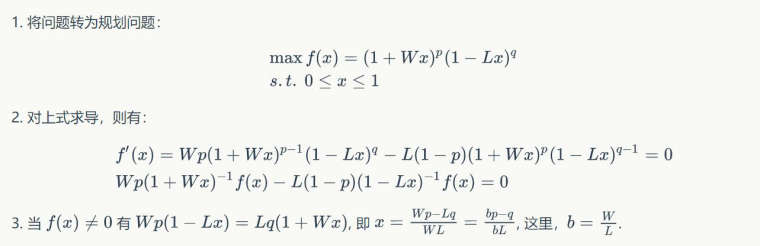掘金小Q 【 论坛管理员 】 发表在策略研究 2018-07-16 11:05:26

3511
1
0• 测试代码

import numpy as np
np.random.seed(1234)
j = 0
for i in range(1000):
results = np.random.randint(0, 2, 1000)
for i in range(1000-7):
if np.all(results[i: i+7] == np.array([1, 1, 1, 1, 1, 1, 1])):
# print(i)
j = j+1
break
print(j)

• 实际输出为 990，也就是说，测试 1000 次，出现连续 7 次正面或以上的次数有990次，也就说说破产概率有 0.99，这样的概率， 一般人估计都承受不了。

init_risk_list = [0.01]
risk_ratio = 1.0
init_cash = 100
gamble_counter = 1000
for init_risk in init_risk_list:
final_risk = init_risk
final_cash = init_cash
for i in range(gamble_counter):
# print(i, risk_ratio, final_cash)
if final_cash <= 0:
# 假如赌博后资金输光，退出循环
print("风险度为 %f，第 %d 次赌博，输光所有" %(init_risk, i))
break
if np.random.randint(0, 2, 1) == 1:
# 赌博赢了，资金变动
final_cash = final_cash + final_cash * final_risk * 1.25
# 如果掷硬币赢了，风险度为初始风险度
final_risk = init_risk
else:
# 赌博输了，资金变动
final_cash = final_cash - final_cash * final_risk
# 如果掷硬币输了，风险度为之前的风险度乘以风险倍率
final_risk = final_risk * risk_ratio
if final_cash > 0:
print("风险度为 %f, 最终资金为 %f"%(init_risk, final_cash))


• 针对鞅策略，不同风险度，经过 1000 次赌博，代码与结果：

# 初始资金
init_cash = 100.0
# 赌博后资金
final_cash = init_cash
# 初始风险
init_risk_list = [0.01, 0.02, 0.03, 0.04, 0.05, 0.1, 0.2, 0.3, 0.4, 0.5]
# 风险倍率
risk_ratio = 2.0
# 赌博次数
gamble_counter = 1000
for init_risk in init_risk_list:
final_risk = init_risk
final_cash = init_cash
for i in range(gamble_counter):
# print(i, risk_ratio, final_cash)
if final_cash <= 0:
# 假如赌博后资金输光，退出循环
print("风险度为 %f，第 %d 次赌博，输光所有" %(init_risk, i))
break
if np.random.randint(0, 2, 1) == 1:
# 赌博赢了，资金变动
final_cash = final_cash + final_cash * final_risk * 1.25
# 如果掷硬币赢了，风险度为初始风险度
final_risk = init_risk
else:
# 赌博输了，资金变动
final_cash = final_cash - final_cash * final_risk
# 如果掷硬币输了，风险度为之前的风险度乘以风险倍率
final_risk = final_risk * risk_ratio
if final_cash > 0:
print("风险度为 %f, 最终风险度为 %f, 最终资金为 %f"%(init_risk, final_risk, final_cash))• 反鞅策略，风险倍率设为 1.0，不同风险度对应的情况

# 初始资金
init_cash = 100.0
# 赌博后资金
final_cash = init_cash
# 初始风险
init_risk_list = [0.01, 0.02, 0.03, 0.04, 0.05, 0.1, 0.2, 0.3, 0.4, 0.5]
# 风险倍率
risk_ratio = 1.0
# 赌博次数
gamble_counter = 1000
for init_risk in init_risk_list:
final_risk = init_risk
final_cash = init_cash
for i in range(gamble_counter):
# print(i, risk_ratio, final_cash)
if final_cash <= 0:
# 假如赌博后资金输光，退出循环
print("风险度为 %f，第 %d 次赌博，输光所有" %(init_risk, i))
break
if np.random.randint(0, 2, 1) == 1:
# 赌博赢了，资金变动
final_cash = final_cash + final_cash * final_risk * 1.25
# 如果掷硬币赢了，风险度为初始风险度
final_risk = init_risk
else:
# 赌博输了，资金变动
final_cash = final_cash - final_cash * final_risk
# 如果掷硬币输了，风险度为之前的风险度乘以风险倍率
final_risk = final_risk * risk_ratio
if final_cash > 0:
print("风险度为 %f, 最终风险度为 %f, 最终资金为 %f"%(init_risk, final_risk, final_cash))《资金管理方法及其应用》 -- 安德烈 昂格尔

•2018-07-23 09:11:16

Looks like your connection to 掘金量化社区 - 量化交易者的交流社区 was lost, please wait while we try to reconnect.

• 提问
遇到问题？到社区提问，我们为您解答.
• 商业
咨询
有商业合作意向？请发邮件联系我们.
• QQ
加入掘金量化QQ群
• 公众号
关注掘金量化微信公众号
• TOP
返回顶部